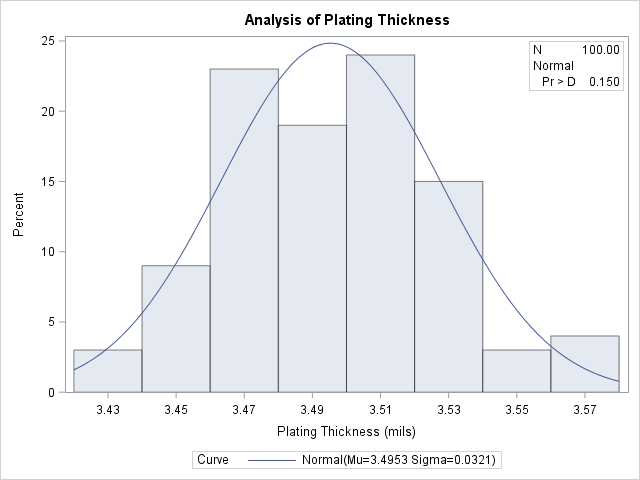# The UNIVARIATE Procedure

### Example 4.19 Adding a Normal Curve to a Histogram

This example is a continuation of Example 4.14. The following statements fit a normal distribution to the thickness measurements in the `Trans` data set and superimpose the fitted density curve on the histogram:

```title 'Analysis of Plating Thickness';
ods select Histogram ParameterEstimates GoodnessOfFit FitQuantiles Bins;
proc univariate data=Trans;
histogram Thick / normal(percents=20 40 60 80 midpercents)
odstitle = title;
inset n normal(ksdpval) / pos = ne format = 6.3;
run;

```

The ODS SELECT statement restricts the output to the "ParameterEstimates," "GoodnessOfFit," "FitQuantiles," and "Bins" tables; see the section ODS Table Names. The NORMAL option specifies that the normal curve be displayed on the histogram shown in Output 4.19.2. It also requests a summary of the fitted distribution, which is shown in Output 4.19.1. goodness-of-fit tests, parameter estimates, and quantiles of the fitted distribution. (If you specify the NORMALTEST option in the PROC UNIVARIATE statement, the Shapiro-Wilk test for normality is included in the tables of statistical output.)

Two secondary options are specified in parentheses after the NORMAL primary option. The PERCENTS= option specifies quantiles, which are to be displayed in the "FitQuantiles" table. The MIDPERCENTS option requests a table that lists the midpoints, the observed percentage of observations, and the estimated percentage of the population in each interval (estimated from the fitted normal distribution). See Table 4.6 for the secondary options that can be specified with after the NORMAL primary option.

Output 4.19.1: Summary of Fitted Normal Distribution

 Analysis of Plating Thickness

The UNIVARIATE Procedure
Fitted Normal Distribution for Thick (Plating Thickness (mils))

Parameters for Normal Distribution
Parameter Symbol Estimate
Mean Mu 3.49533
Std Dev Sigma 0.032117

Goodness-of-Fit Tests for Normal Distribution
Test Statistic p Value
Kolmogorov-Smirnov D 0.05563823 Pr > D >0.150
Cramer-von Mises W-Sq 0.04307548 Pr > W-Sq >0.250
Anderson-Darling A-Sq 0.27840748 Pr > A-Sq >0.250

Histogram Bin Percents
for Normal Distribution
Bin
Midpoint
Percent
Observed Estimated
3.43 3.000 3.296
3.45 9.000 9.319
3.47 23.000 18.091
3.49 19.000 24.124
3.51 24.000 22.099
3.53 15.000 13.907
3.55 3.000 6.011
3.57 4.000 1.784

Quantiles for Normal Distribution
Percent Quantile
Observed Estimated
20.0 3.46700 3.46830
40.0 3.48350 3.48719
60.0 3.50450 3.50347
80.0 3.52250 3.52236

Output 4.19.2: Histogram Superimposed with Normal CurveThe histogram of the variable `Thick` with a superimposed normal curve is shown in Output 4.19.2.

The estimated parameters for the normal curve ( and ) are shown in Output 4.19.1. By default, the parameters are estimated unless you specify values with the MU= and SIGMA= secondary options after the NORMAL primary option. The results of three goodness-of-fit tests based on the empirical distribution function (EDF) are displayed in Output 4.19.1. Because the p-values are all greater than 0.15, the hypothesis of normality is not rejected.

A sample program for this example, uniex08.sas, is available in the SAS Sample Library for Base SAS software.